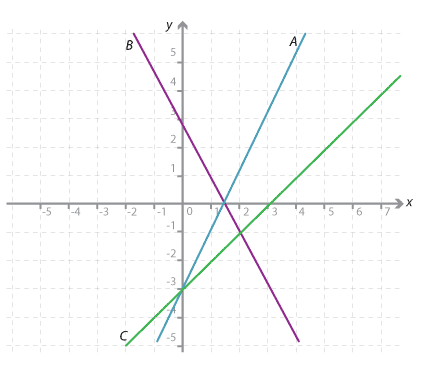#### Question 2

For the rule y = −2x + 3 complete the table of values.

 x y –3 –2 –1 0 1 2 3 9 7 5 x axis is 0, what is y axis? x axis is 1, what is y axis? −1 −3

Please fill in both values before checking the answer.

#### Question 3

Which of the following straight lines is the graph of y = −2x + 3?Detailed description

 A B C